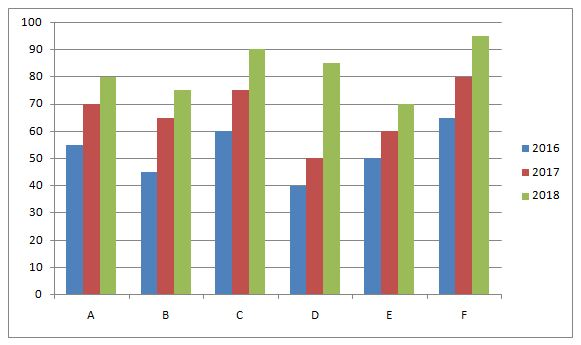# NIACL AO Mains– Quantitative Aptitude Questions Day- 24

Dear Readers, Bank Exam Race for the Year 2019 is already started, To enrich your preparation here we have providing new series of Practice Questions on Quantitative Aptitude – Section. Candidates those who are preparing for NIACL AO Mains 2019 Exams can practice these questions daily and make your preparation effective.

[WpProQuiz 5332]

Direction (1- 5): Find the wrong term in the following number series?

1) 324, 476, 712, 988, 1289

a) 712

b) 988

c) 1289

d) 476

e) 324

2) 2400, 1200, 800, 600, 460, 400

a) 460

b) 800

c) 1200

d) 600

e) 400

3) 420, 2516, 504, 2016, 672, 1344

a) 504

b) 1344

c) 672

d) 2516

e) 2016

4) 33, 16, 17, 24, 50, 123, 370.5

a) 24

b) 123

c) 370.5

d) 50

e) 33

5) 360, 1036, 460, 944, 503, 868

a) 1036

b) 944

c) 503

d) 868

e) 360

Directions (Q. 6 – 10): Study the following information carefully and answer the given questions:

The following bar graph shows the total number of newspaper readers (In lakhs) of 6 different states in 3 different years.6) Find the difference between the total number of male newspaper readers of state C in the year 2017 to that of total number of female newspaper readers of state D in the year 2018, if the ratio between the male to that of female newspaper readers of state C in the year 2017 and that of state D in the year 2018 is 8 : 7 and 10 : 7 respectively?

a) 2 lakhs

b) 8 lakhs

c) 5 lakhs

d) 3 lakhs

e) None of these

7) Find the ratio between the total number of newspaper readers in the year 2016 to that of 2018 in all the given states together?

a) 7: 11

b) 8: 13

c) 21: 32

d) 123: 157

e) None of these

8) Total number of newspaper readers of State B in all the given years together is approximately what percentage of total number of newspaper readers of State E in all the given years together?

a) 87 %

b) 115 %

c) 72 %

d) 103 %

e) 66 %

9) Find the average number of newspaper readers from state G, H and D together in all the given years, if the total number of newspaper readers from state G and H in all the given years is 20 % more than the total number of newspaper readers from state E and 20 % less than the total number of newspaper readers from state E respectively?

a) 196.66 lakhs

b) 178.33 lakhs

c) 180.5 lakhs

d) 175 lakhs

e) None of these

10) In which state has second lowest number of newspaper readers in all the given years together?

a) State A

b) State C

c) State E

d) State D

e) State B

Directions (Q. 1 – 5):

The correct series is,

324             476             712             990             1289

152             236             278             299

84               42               21

The difference of difference is, ÷ 2

The wrong term is, 988

The correct series is,

2400, 1200, 800, 600, 480, 400

The pattern is, *1/2, *2/3, *3/4, *4/5, *5/6

The wrong term is, 460

The correct series is,

420, 2520, 504, 2016, 672, 1344

The pattern is, *6, ÷ 5, *4, ÷ 3, *2,…

The wrong term is, 2516

The correct series is,

33, 16, 17, 24, 50, 122.5, 370.5

The pattern is, *0.5 – 0.5, *1 + 1, *1.5 – 1.5, *2 + 2, *2.5 – 2.5, *3 + 3,…

The wrong term is, 123

The correct series is,

360, 1036, 460, 944, 544, 868

The pattern is, +262, -242, +222, -202, +182,…

The wrong term is, 503

Directions (Q. 6 – 10):

The total number of male newspaper readers of state C in the year 2017

= > (75/15)*8 = 40 lakhs

The total number of female newspaper readers of state D in the year 2018

= > (85/17)*7 = 35 lakhs

Required difference = 40 – 35 = 5 lakhs

The total number of newspaper readers in the year 2016 in all the given states together

= > 55 + 45 + 60 + 40 + 50 + 65 = 315 lakhs

The total number of newspaper readers in the year 2018 in all the given states together

= > 80 + 75 + 90 + 85 + 70 + 95 = 495 lakhs

Required ratio = 315: 495 = 7: 11

Total number of newspaper readers of State B in all the given years together

= > 45 + 65 + 75 = 185 lakhs

Total number of newspaper readers of State E in all the given years together

= > 50 + 60 + 70 = 180 lakhs

Required % = (185/180)*100 = 102.77 = 103 %

The total number of newspaper readers from state G, H and D together in all the given years

= > 180*(120/100) + 180*(80/100) + 175

= > 216 + 144 + 175 = 535 lakhs

Required average = 535/3 = 178.33 lakhs

State A = > 55 + 70 + 80 = 205 lakhs

State B = > 45 + 65 + 75 = 185 lakhs

State C = > 60 + 75 + 90 = 225 lakhs

State D = > 40 + 50 + 85 = 175 lakhs

State E = > 50 + 60 + 70 = 180 lakhs

State F = > 65 + 80 + 95 = 240 lakhs

State E has second lowest number of newspaper readers.